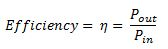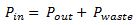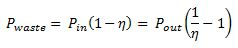# Tutorial: Power Supply Efficiency

This tutorial installment is: Power Supply Efficiency. This topic answers the following questions:

• What is power supply efficiency and how is it defined?
• How is power supply efficiency defined?
• What are the effects of efficiency on power converter design?

Last topic: Linear Supplies
Next topic: Power Supply Capacitors and Inductors

## Power Supply Efficiency: Introduction

Efficiency is typically thought of as a measure of how well a product performs its task without waste. When waste is reduced through improved efficiency, there is typically less cost. In the case of a converter, efficiency is a measure of how much power at the input appears at the output, and therefore less waste. Obviously, it is important, and considered technologically “green”, to conserve energy, especially in the case where a battery is the source of energy. However, efficiency, as applied to electronic power supplies, has a far more important aspect than just conserving energy. Power supply efficiency has a direct effect upon the upper limit of output power given a package size and mode of cooling. This consideration often dominates the design of a converter, especially since there is almost always a drive toward size reduction and miniaturization of electronics products.

## Power Supply Efficiency: Definition

Very simply put, power supply efficiency is the output power divided by the input power:## Effects of Efficiency in Power Converter Design

The Law of Conservation of Energy is always observed. Therefore the energy that goes into a power converter must equal the energy that comes out plus some waste energy. This waste energy normally is in the form of heat. Power in and out of a power converter obeys the following relationship:In terms of efficiency, the waste heat can be expressed as:Poor efficiency means greater waste heat to manage. To illustrate the importance of efficiency, consider the following example. Two DC/DC converters are operated at room temperature, each processing 200W at the output. Each converter is in the same size package which has a thermal resistance to heat transfer of 2 deg C per watt. Converter A operates at a state of the art efficiency of 95%. Converter B operates at an efficiency of 80%. The waste power for converter A operating at 95% efficiency would be about 200W * (1 / 0.95 – 1)=10.53W. At room temperature, with a case to ambient thermal resistance of 2C/W, the case temperature would be about 46C, cool enough to hold in the palm of your hand while processing 200W. The waste power for converter B operating at 80% efficiency would be about 200W * ( 1 / 0.80 – 1) = 50W. At the same room temperature and the same case to ambient thermal resistance, the case temperature would be about 125C, hotter than boiling water and hotter than many electronic components are rated for! To solve the problem of excessive temperature rise, one or more of the following actions must happen:

• The efficiency must to increased to lessen the waste energy which is dissipated as heat,
• The package size must be increased to provide more surface area for heat transfer which reduces the case to ambient thermal resistance,
• Active and/or conduction cooling techniques must be employed,
• The processed power must be reduced.

The first choice would be to improve efficiency as the other choices are usually not desirable. Thus efficiency is a vital measure to converter performance. Any generated waste heat must be removed by a heat transfer method which keeps the component temperatures within a range for acceptable reliability. If efficiency can be kept high, then the burden of waste heat transfer methods to keep the component temperatures in an acceptable temperature range is much less. Linear supplies with low efficiency must have larger package sizes, or forced cooling, or lower power output. Whereas, switching supplies which typically have efficiencies starting at around 80% can enjoy smaller package sizes, perhaps no forced cooling, and higher power outputs.

## Next Topic

The next tutorial installment is: Power Supply Capacitors and Inductors. This next topic will answer the following questions:

• What is the purpose of capacitors and inductors in power electronics?
• What are the energy storage and terminal time properties of capacitors and inductors?
• What are the power supply applications of capacitors and inductors?

If you need assistance with power electronics design, call or email us today for help with your requirements. You can also go to our power electronics consultant website for more information about our services for business clients. Thank you for reading this tutorial article entitled “Power Supply Efficiency”

Next topic: Power Supply Capacitors and Inductors